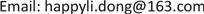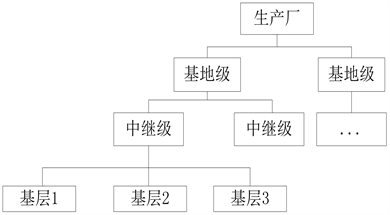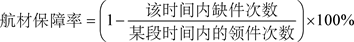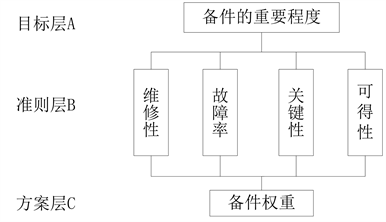﻿ 基于可靠性分析的某型航空发动机备件需求分析 Requirement Analysis of Spare Parts for Aero-Engine Based on Reliability Analysis

Dynamical Systems and Control
Vol. 09  No. 01 ( 2020 ), Article ID: 33274 , 9 pages
10.12677/DSC.2020.91001

Requirement Analysis of Spare Parts for Aero-Engine Based on Reliability Analysis

Li Ma1, Dong Li2, Yubo Feng3, Tao Sun4

1The Second Military Representative Office of the Naval Equipment Department in Shenyang, Shenyang Liaoning

2Unit 91899, Huludao Liaoning

3Unit 92728, Shanghai

4Naval Aviation University, Yantai ShandongReceived: Nov. 14th, 2019; accepted: Nov. 27th, 2019; published: Dec. 4th, 2019ABSTRACT

This paper mainly analyzes the inherent reliability of a certain engine. The fault data of its test stage are processed. Using the method of solving of fusion matrix and spare parts optimal model, combined with the data of components and the whole machine, this paper analyzes the reliability of the whole machine. Finally, the guaranteed rate of a single engine is 90%, the minimum cost is 681,700 yuan, the corresponding number of spare parts and the weight of each factor affecting the importance of spare parts are gotten.

Keywords:Reliability Analysis, Aeroengine, Spare Parts Demand

1海军装备部驻沈阳地区第二军事代表室，辽宁 沈阳

291899部队，辽宁 葫芦岛

392728部队，上海

4海军航空大学，山东 烟台1. 引言

2. 备件供应工作内容

2.1. 备件目录种类

1) 随机备件目录：主要以在飞机的保证期内，用于恢复飞机主机、机载成品与地面保障设备的效能所需的不可修复件和部分可修复件为主要内容。不可修复件包括消耗性备件和易损性备件。可修备件主要指飞机维修性所划定的一些外场可更换件。

2) 推荐订货备件目录：国外亦称为后续备件目录，是由承包商向用户推荐的订货备件目录，以用于保证期后(包括部分保证期内的不属于随机备件范围内的备件)恢复系统效能和保证飞机持续使用所需的备件为主要内容。其由使用方订货，费用不计入飞机成本。

2.2. 备件供应体制Figure 1. Place of supply of spare parts

3. 备件需求量计算方法

3.1. 备件需求量计算模型

$\left\{\begin{array}{l}\mathrm{min}\underset{i=1}{\overset{n}{\sum }}{c}_{i}{x}_{i},i=1,2,\cdots ,n\\ \underset{i=1}{\overset{n}{\prod }}{R}_{i}\left({x}_{i},{t}_{i}\right)>{R}_{0}\end{array}$ (1)(2)

${R}_{i}=P\left\{{N}_{i}\left(t\right)\le {x}_{i}\right\}$ (3)

${R}_{s}=P\left\{{N}_{1}\left(t\right)+{N}_{2}\left(t\right)+\cdots +{N}_{n}\left(t\right)\le \left({x}_{1}+{x}_{2}+\cdots +{x}_{n}\right)\right\}$ (4)

${x}_{i}^{0}=\left\{\begin{array}{l}{x}_{i},{N}_{i}\left(t\right)\ge {x}_{i}\\ {N}_{i}\left(t\right),{N}_{i}\left(t\right)<{x}_{i}\end{array}$ (5)

${\stackrel{^}{R}}_{s}=\frac{\underset{i=1}{\overset{n}{\sum }}{x}_{i}^{0}}{\underset{i=1}{\overset{n}{\sum }}{N}_{i}\left(t\right)}=\underset{i=1}{\overset{n}{\sum }}\frac{{N}_{i}\left(t\right)}{\underset{i=1}{\overset{n}{\sum }}{N}_{i}\left(t\right)}{R}_{i}$ (6)

${R}_{s}=\underset{i=1}{\overset{n}{\sum }}\frac{{\lambda }_{i}}{\underset{i=1}{\overset{n}{\sum }}{\lambda }_{i}}{R}_{i}$ (7)

${\rho }_{i}=\frac{{\lambda }_{i}}{\underset{i=1}{\overset{n}{\sum }}{\lambda }_{i}},i=1,2,\cdots ,n$

${R}_{s}=\underset{i=1}{\overset{n}{\sum }}{\rho }_{i}{R}_{i}$ (8)

1) 基于一定经费的后续备件保障模型的目标函数为

$R=\mathrm{max}\underset{i=1}{\overset{N}{\sum }}\rho \left(i\right)R\left(i\right)$ (9)

$C=\underset{i=1}{\overset{N}{\sum }}C\left(i\right)X\left(i\right)\le C\left(0\right)$ (10)

$C\left(i\right)$ 为第i个备件的单价，式10表示对各部件的数量和单价的乘积进行求和并且使其值小于或等于系统总经费 $C\left(0\right)$(11)

$R\left(00\right)\le R\left(X\left(i\right),T\right),i=1,2,\cdots ,k,R\left(01\right)\le R\left(00\right)\le R\left(02\right)$ (12)

3.2. 基于保障率的后续备件保障模型

$C=\mathrm{min}\underset{i=1}{\overset{N}{\sum }}C\left(i\right)X\left(i\right)$ (13)

$R\left(01\right)\le R\left(X\left(i\right),T\right)\le R\left(02\right)$ (14)

$\underset{i=1}{\overset{N}{\sum }}\rho \left(i\right)R\left(i\right)\ge R\left(0\right)$ (15)

$\underset{i=1}{\overset{J-1}{\sum }}\rho \left(i\right)R\left(i\right)+\underset{i=J+1}{\overset{N}{\sum }}\rho \left(i\right)R\left(i\right)+\rho \left(J\right)×R\left(X\left(J\right)-1,T\right)\le R\left(0\right)$ (16)

J为 $1,\cdots ,N$ 中的任一数值。表示当第J个备件缺一个时系统总保障率R小于 $R\left(0\right)$

3.3. 优化保障模型

1) 构造判断矩阵Figure 2. Weight hierarchy chart

${a}_{ij}=\frac{1}{{a}_{ji}},{a}_{ii}=1,\left(i,j=1,2,\cdots ,n\right)$ (17)

${a}_{ij}=\left[{a}_{ij}^{-},{a}_{ij}^{+}\right]=\left\{\begin{array}{l}\left[{a}_{ij}-\delta ,{a}_{ij}+\delta \right]\left({a}_{ij}\ge 1,i\ne j\right)\\ \left[\frac{1}{1/{a}_{ij}+\delta },\frac{1}{1/{a}_{ij}-\delta }\right]\left({a}_{ij}\le 1,i\ne j\right)\\ \left[1,1\right]\left(i=j\right)\end{array}$ (18)

${a}_{ij}$ 为模糊判断矩阵中的元素，因此判断矩阵 ${A}^{\left(k\right)}$ 为正互反矩阵。

2) 计算归一化权重向量

$\alpha ={\left[\underset{j=1}{\overset{n}{\sum }}\frac{1}{\underset{i=1}{\overset{n}{\sum }}{a}_{ij}^{+}}\right]}^{1/2}$ , $\beta ={\left[\underset{j=1}{\overset{n}{\sum }}\frac{1}{\underset{i=1}{\overset{n}{\sum }}{a}_{ij}^{-}}\right]}^{1/2}$ (19)

$C.I.=\frac{\left(\alpha {\lambda }_{\mathrm{max}}^{-}+\beta {\lambda }_{\mathrm{max}}^{+}\right)/2-n}{n-1}$ (20)

$C.I.\le 0.1$，就证明判断矩阵是一致的。

3) 计算决策权重

$w=\frac{1}{\underset{k=1}{\overset{k}{\sum }}{y}_{k}}\underset{k=1}{\overset{k}{\sum }}{y}_{k}{w}_{0}^{\left(k\right)}$ (21)

4) 部件的最终权重

4. 某型发动机备件方案分析

4.1. 备件权重

${A}^{\left(1\right)}=\left[\begin{array}{cccc}\left[1,1\right]& \left[\frac{1}{6},\frac{1}{4}\right]& \left[\frac{1}{4},\frac{1}{2}\right]& \left[4.5,5.5\right]\\ \left[4,6\right]& \left[1,1\right]& \left[\frac{1}{6},\frac{1}{4}\right]& \left[1.5,4.5\right]\\ \left[2,4\right]& \left[4,6\right]& \left[1,1\right]& \left[4,6\right]\\ \left[\frac{1}{5.5},\frac{1}{4.5}\right]& \left[\frac{1}{4.5},\frac{1}{1.5}\right]& \left[\frac{1}{6},\frac{1}{4}\right]& \left[1,1\right]\end{array}\right]$

${A}^{\left(2\right)}=\left[\begin{array}{cccc}\left[1,1\right]& \left[\frac{1}{8.5},\frac{1}{5.5}\right]& \left[\frac{1}{4},\frac{1}{2}\right]& \left[4,6\right]\\ \left[5.5,8.5\right]& \left[1,1\right]& \left[\frac{1}{4},\frac{1}{2}\right]& \left[4,6\right]\\ \left[2,4\right]& \left[2,4\right]& \left[1,1\right]& \left[5.5,8.5\right]\\ \left[\frac{1}{6},\frac{1}{4}\right]& \left[\frac{1}{6},\frac{1}{4}\right]& \left[\frac{1}{8.5},\frac{1}{5.5}\right]& \left[1,1\right]\end{array}\right]$

${x}^{-\left(1\right)}=\left(\text{0}\text{.2424,0}\text{.2172,0}\text{.2172,0}\text{.1018}\right)$

${x}^{+\left(1\right)}=\left(\text{0}\text{.2363,0}\text{.2738,0}\text{.2738,0}\text{.0767}\right)$

${w}^{\left(k\right)}=\left(\alpha {x}^{-}+\beta {x}^{+}\right)/2$ 计算权重向量并进行归一化得专家1的权重分配 ${w}^{0\left(1\right)}=\left[\text{0}\text{.2915,0}\text{.3000,0}\text{.3000,0}\text{.1085}\right]$。由式20计算 $C.I.=0.078$ 满足一致性要求。

4.2. 优化备件保障方案

5. 总结

Requirement Analysis of Spare Parts for Aero-Engine Based on Reliability Analysis[J]. 动力系统与控制, 2020, 09(01): 1-9. https://doi.org/10.12677/DSC.2020.91001

1. 1. Wu, Y.T., Enright, M.P., Millwater, H.R., et al. (2000) Leverant. Probabilistic Methods for Design Assessment of Reliability with Inspection (DARWINTM). AIAA-2000-1510.
https://doi.org/10.2514/6.2000-1510

2. 2. 孙伟, 高连华, 曹玉坤, 等. 装甲车辆可靠性理论与应用[M]. 北京: 兵器工业出版社, 2006: 20.

3. 3. 黄俊. 基于可靠性的民机备件支援及其软件系统[D]: [博士学位论文]. 南京: 南京航空航天大学, 2006.

4. 4. 姜柏松, 孙春林. 一种改进的备件系统优化模型及解法[J]. 中国民航学院学报, 2003, 21(6): 17-20.

5. 5. Barlow, R.H. (2004) Business Logistics Management: Planning Organizing and Controlling the Supply Chain. Pearson Education, Inc., Upper Saddle River, New Jersey.

6. 6. 孙进康, 郦正能. 可修复系统故障数据分析模型与方法研究[J]. 解放军理工大学学报, 2000(2): 57-62.

7. 7. 孙有朝. 复杂系统可靠性多级综合的熵法第二近似限[J]. 控制与决策, 2002, 17(4): 423-426.

8. 8. 周源泉, 翁朝曦. 可靠性评定[M]. 北京: 科学出版社, 1990: 37-40.